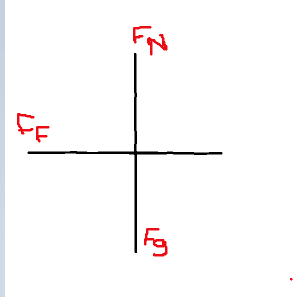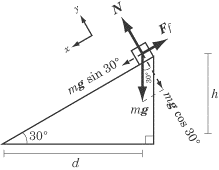# How to solve for coefficient of static friction with mass

Its for my final project. We are doing a sliding a textbook down an *incline plane* and we are expected to find coefficient of static friction without using newton guage, and we are also now allowed to use kinematics. I just need some infomration on how to do this, like a formula or something in that sort, thanks in advance. I need this before tonight, so anything after 9pm wouldn't be nessecary as i would be sleeping :P thanks in advance

## Homework Equations

Fs = UsN but we dont have force..

## The Attempt at a Solution

no attempt yet

berkeman
Mentor
Its for my final project. We are doing a sliding a textbook down an *incline plane* and we are expected to find coefficient of static friction without using newton guage, and we are also now allowed to use kinematics. I just need some infomration on how to do this, like a formula or something in that sort, thanks in advance. I need this before tonight, so anything after 9pm wouldn't be nessecary as i would be sleeping :P thanks in advance

## Homework Statement

2. Homework Equations
Fs = UsN but we dont have force..

## The Attempt at a Solution

no attempt yet [/B][/B]
Welcome to the PF.

Start by drawing a free body diagram (FBD) of the book on the inclined plane. Label all the forces on the book. Then find the angle where the book just starts to slip. Hint -- the mass of the book will end up canceling out of the equations... Please show us your FBD and your calculations...haruspex
Homework Helper
Gold Member
2020 Award
Its for my final project. We are doing a sliding a textbook down an *incline plane* and we are expected to find coefficient of static friction without using newton guage, and we are also now allowed to use kinematics. I just need some infomration on how to do this, like a formula or something in that sort, thanks in advance. I need this before tonight, so anything after 9pm wouldn't be nessecary as i would be sleeping :P thanks in advance

## Homework Statement

2. Homework Equations
Fs = UsN but we dont have force..

## The Attempt at a Solution

no attempt yet [/B][/B]
I don't know what timezone you are in, so I cannot tell how much time you have. Anyway, you do need to post complete information (what is the equipment set-up? What can you measure?) and show some attempt, even if it is just some thoughts.
Do you know how to draw a free body diagram? What relationship do you know between force and acceleration?

See I have no calculations because I just dont understand what we are supposed to do with *JUST MASS* , I searched it up and realized a lot of people talking about angles and stuff. Can you please (not teach me) but inform me on the basics on how to use mass to find static coefficient. Here is the fbd assuming its accelerating eastwardsI don't know what timezone you are in, so I cannot tell how much time you have. Anyway, you do need to post complete information (what is the equipment set-up? What can you measure?) and show some attempt, even if it is just some thoughts.
Do you know how to draw a free body diagram? What relationship do you know between force and acceleration?

Like I said, we have a incline plane, and we are putting a textbook ontop of it and letting it go down. We are given the mass of the textbook. We are not allowed to use kinematics or a newton guage, so all I have is mass. I am supposed to find the Coefficient of static friction of the textbook on the incline plane. I know how to make a FBD (as shown above), and I know that Fnet = ma, and I know how to setup fnet equations. I just dont understand what I can do with this single mass given to me to find the coefficient.

I am eastern time zone, and thanks for replying

berkeman
Mentor
See I have no calculations because I just dont understand what we are supposed to do with *JUST MASS* , I searched it up and realized a lot of people talking about angles and stuff. Can you please (not teach me) but inform me on the basics on how to use mass to find static coefficient. Here is the fbd assuming its accelerating eastwards
That drawing does not show an inclined plane. It is flat, not inclined.

Try a Google Images search on mass on inclined plane with friction, and you will get lots of examples of FBDs for this problem. The book will just start to slip when the force of friction pointing back up the inclined plane (parallel to the inclined plane surface) is equal to the force of gravity on the book pointing down the inclined plane (parallel to the plane). Please look at the forces labeled on the Google Images FBDs and write the equation that equates the forces that I just mentioned.That drawing does not show an inclined plane. It is flat, not inclined.

Try a Google Images search on mass on inclined plane with friction, and you will get lots of examples of FBDs for this problem. The book will just start to slip when the force of friction pointing back up the inclined plane (parallel to the inclined plane surface) is equal to the force of gravity on the book pointing down the inclined plane (parallel to the plane). Please look at the forces labeled on the Google Images FBDs and write the equation that equates the forces that I just mentioned.Haha , yeah I know its flat in the picture, juts image it wasnt flat, I didnt wanna spend too much time rotating it and stuff ( I have exams ) and, ill give it a go.

Fnet = Fn - Ff - Fg
ma = mg - μmg - mg? sorry its been 2 months since ive done forces unit, but im assuming fg and fn cancel each other out, and we are left with Fnet = Ff?

berkeman
Mentor
Haha , yeah I know its flat in the picture, juts image it wasnt flat, I didnt wanna spend too much time rotating it and stuff ( I have exams ) and, ill give it a go.

Fnet = Fn - Ff - Fg
ma = mg - μmg - mg? sorry its been 2 months since ive done forces unit, but im assuming fg and fn cancel each other out, and we are left with Fnet = Ff?
You should be showing a FBD like this one, and setting the forces equal that I mentioned...

http://img.sparknotes.com/content/testprep/bookimgs/sat2/physics/0008/incline_withfFBD.gifYou should be showing a FBD like this one, and setting the forces equal that I mentioned...

http://img.sparknotes.com/content/testprep/bookimgs/sat2/physics/0008/incline_withfFBD.gifSo what I am learning is that I need to get the angle.

Like I said I watched a video earlier and he said you do Us = tan30, that seems very odd.

The formula I have familiar with is Us = F/mg but we dont have force.

haruspex
Homework Helper
Gold Member
2020 Award
So what I am learning is that I need to get the angle.

Like I said I watched a video earlier and he said you do Us = tan30, that seems very odd.

The formula I have familiar with is Us = F/mg but we dont have force.
##\mu_s=\tan(\theta)##, where theta is the angle of the slope, applies in a particular circumstance. It is not generally true.
Neither the mass nor the exact force are of interest, only the ratio between them. If you were to double the mass, all the forces would double.

The force formula you quote is not necessarily correct. You need to say exactly what F represents there.

Since the task is in relation to static friction, the exact acceleration is not interesting either: you just have to distinguish between some acceleration and none.

Getting the diagram right is important. Showing it as level ground led to your mistaken belief that mg and the normal force would cancel.

##\mu_s=\tan(\theta)##, where theta is the angle of the slope, applies in a particular circumstance. It is not generally true.
Neither the mass nor the exact force are of interest, only the ratio between them. If you were to double the mass, all the forces would double.

The force formula you quote is not necessarily correct. You need to say exactly what F represents there.

Since the task is in relation to static friction, the exact acceleration is not interesting either: you just have to distinguish between some acceleration and none.

Getting the diagram right is important. Showing it as level ground led to your mistaken belief that mg and the normal force would cancel.

I gave you all I got, thats what we learned this year. I dont understand how I can solve for this Us, if just given the mass (and I can calculate the angle). I would assume, it has to do with Fg, Fn, and the angle (and of course mass)

haruspex
Homework Helper
Gold Member
2020 Award
I gave you all I got, thats what we learned this year. I dont understand how I can solve for this Us, if just given the mass (and I can calculate the angle). I would assume, it has to do with Fg, Fn, and the angle (and of course mass)
As I said, forget the mass. You may be given it but it is not relevant. Just call it m.
With the right experiment, all that matters is the angle. Are you told what experiment to conduct, or are you supposed to figure that out?
Look at berkemen's diagram. What are the forces acting parallel to the slope? (Do you know how to find the component of a force in a given direction in relation to the force?). What are the forces acting normally to the slope? In a static situation, what equations relate these forces?

The experiment is, we put a textbook on an incline and let it go down. "find the Us"

The only force that is parallel is "Ff" , and Fg + Fn are perpendicular.

The only formula we have learned relating to this is Fsmax = Fn/Fg or Fnet = Fn - Ff - Fg like I mentioned earlier.

haruspex
Homework Helper
Gold Member
2020 Award
The only force that is parallel is "Ff" , and Fg + Fn are perpendicular.
No, mg is not perpendicular to the slope. Take another look at berkeman's diagram.
The only formula we have learned relating to this is Fsmax = Fn/Fg or Fnet = Fn - Ff - Fg like I mentioned earlier.
Equations don't mean anything unless you specify what the variables represent. On the face of it, neither of those equations makes any sense.
The first one appears to have a force equalling a force divided by another force.
The second has you taking the difference between two forces (Fn, Ff) that are at right angles to each other.
Here's a correct equation that might help:
##F_{f,max}=\mu_s F_n##, where ##\mu_s## is the coefficient of static friction, ##F_n## is the normal force between the surfaces, and ##F_{f,max}## is the maximum frictional force, i.e., the frictional force that exists just before the object slides.
The experiment is, we put a textbook on an incline and let it go down. "find the Us"
that will not give you the static coefficient. If you were to measure the acceleration as it slides down you could find the kinetic coefficient that way.
To find the static coefficient you must gradually increase the angle of the slope until the book starts to slide. From that critical angle you can find the static coefficient.